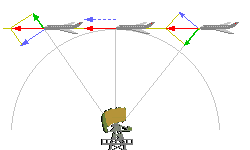# RV

Radial velocity##### Radial velocity

The radial velocity of an object with respect to a given point is the rate of change of the distance between the object and the point. That is, the radial velocity is the component of the object's velocity that points in the direction of the radius connecting the point and the object. In astronomy, the point is usually taken to be the observer on Earth, so the radial velocity then denotes the speed with which the object moves away from the Earth.

License Image-Source